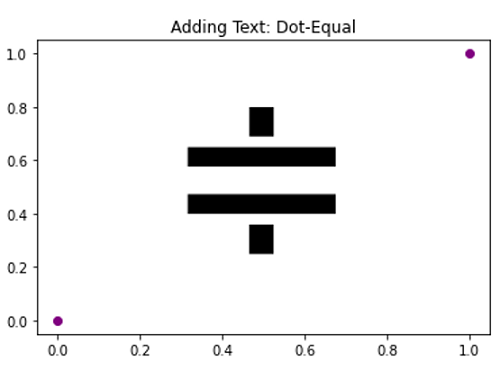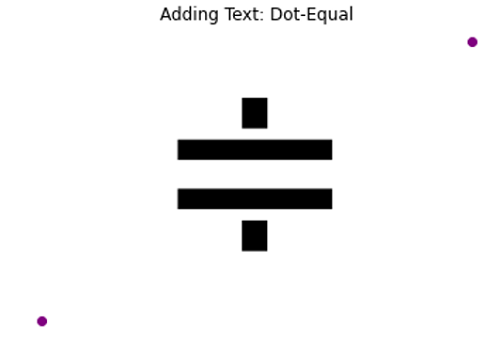# Dot-Equal Symbol in Python Plotting

Here, we are going to learn how to add dot-equal symbol in Python plotting?
Submitted by Anuj Singh, on September 11, 2020

Dot-Equal is a different symbol used in set theory and other mathematical expressions. Python has provided us to use it while plotting.

Following example shows its implementation.

```plt.text(0.25, 0.25, r'\$\Doteq\$', fontsize=175)
```

Illustrations:## Python code for dot-equal symbol in plotting

```import numpy as np
import matplotlib.pyplot as plt

# Dot-Equal
# In text
plt.figure()
plt.plot([0,1],[0,1], 'o', color=  'purple')
plt.text(0.25, 0.25, r'\$\Doteq\$', fontsize=175, )
plt.show()

plt.figure()
plt.plot([0,1],[0,1], 'o', color=  'purple')
plt.text(0.25, 0.25, r'\$\Doteq\$', fontsize=175, )
plt.axis(False)
plt.show()
```

Output:

```Output is as Figure
```